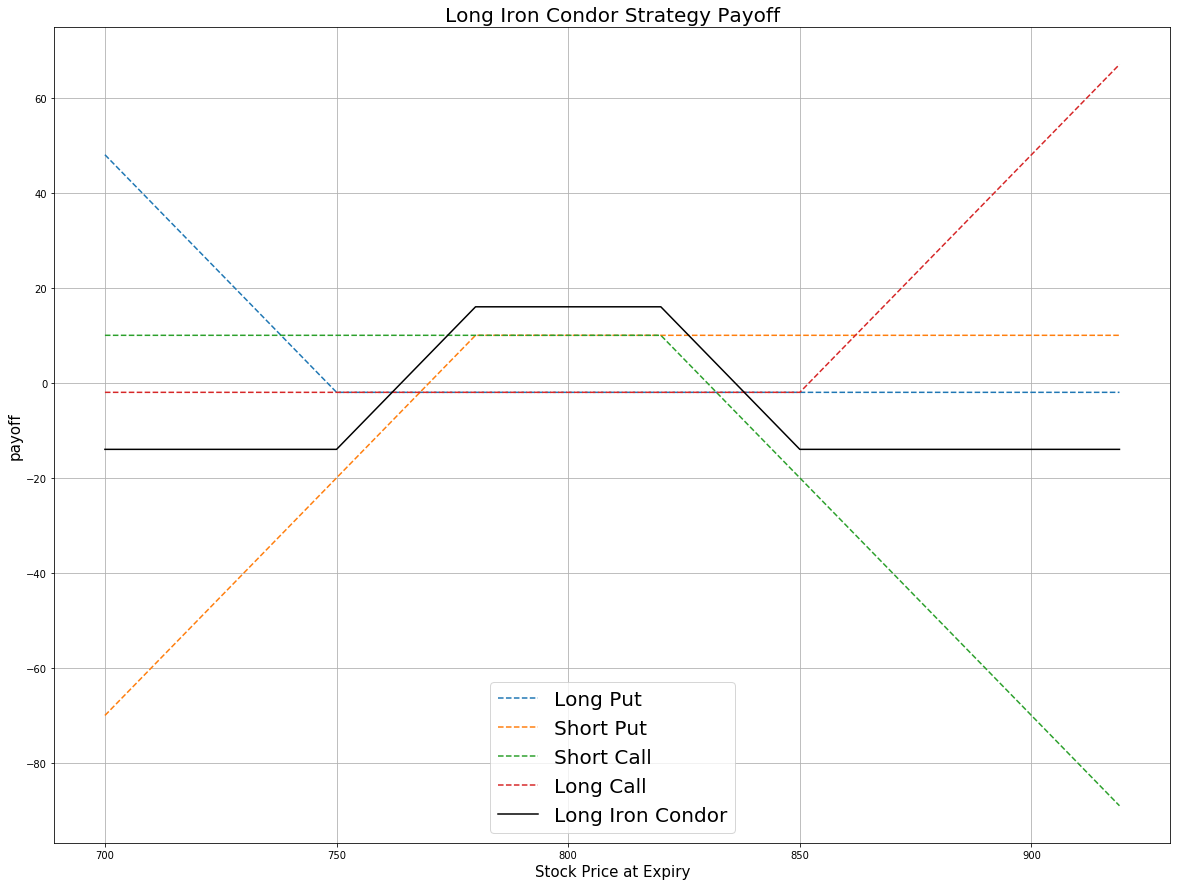### Definition

An Iron Condor is an option strategy which involves four option contracts. All options have the same expiration date but different strike prices, where generally the spread in the put strike prices is the same as the spread in the calls. The order of strike for four contracts is A > B > C > D.

Position Strike
long 1 OTM put  A
short 1 OTM put  B
short 1 OTM call  C
long 1 OTM call  D

The Iron Condor is the combination of a bear put spread and a bull call spread in which the strike price of the long put is lower than the strike price of the long call. If the stock price is between the two short strike prices when the options expire, the strategy will be profitable.

price = np.arange(700,920,1)
k_call_higher = 850 # the strike price of OTM call(Higher k)
k_call_lower = 820 # the strike price of OTM call(Lower k)
k_put_higher = 780 # the strike price of OTM put(Higher k)
k_put_lower = 750 # the strike price of OTM put(Lower k)
# payoff for the long put position
# payoff for the short put position
# payoff for the short call position
# payoff for the long call position
# payoff for Long Iron Condor Strategy
payoff = np.sum([payoff_long_put,payoff_short_put,payoff_short_call,payoff_long_call], axis=0)
plt.figure(figsize=(20,15))
plt.plot(price, payoff_long_put, label = 'Long Put',linestyle='--')
plt.plot(price, payoff_short_put, label = 'Short Put',linestyle='--')
plt.plot(price, payoff_short_call, label = 'Short Call',linestyle='--')
plt.plot(price, payoff_long_call, label = 'Long Call',linestyle='--')
plt.plot(price, payoff, label = 'Long Iron Condor',c='black')
plt.legend(fontsize = 20)
plt.xlabel('Stock Price at Expiry',fontsize = 15)
plt.ylabel('payoff',fontsize = 15)
plt.title('Long Iron Condor Strategy Payoff',fontsize = 20)
plt.grid(True)Here the strike price is A (750), B (780), C (820) and D (850). As seen in the payoff plot, the maximum profit comes from the options premium because the deeper OTM options are cheaper than the shallower ones. It is reached when the stock price is between the higher put strike and lower call strike (the two short positions) and the options all expire worthless. The maximum loss of Iron Condor is reached when the stock price is lower than the lowest strike A, then the two call options become worthless, the two puts are in the money. For the other condition, the stock price is higher than the highest strike D. Consequently, the two call options are in the money but the two puts expire worthless.

### Implementation

Step 1: Initialize your algorithm by setting the start date, the end date and the cash for your algorithm. Then, implement the coarse selection of option contracts.

def Initialize(self):
self.SetStartDate(2017, 2, 1)
self.SetEndDate(2017, 3, 1)
self.SetCash(500000)
self.symbol = option.Symbol
option.SetFilter(self.UniverseFunc)
# use the underlying equity GOOG as the benchmark
self.SetBenchmark(equity.Symbol)

def UniverseFunc(self, universe):
return universe.IncludeWeeklys().Strikes(-15, 15).Expiration(0, 40)


Step 2: Break the candidate options into two parts: calls and puts.

for i in optionchain:
if i.Key != self.symbol: continue
chain = i.Value
# filter the call and put options on the contracts
call = [i for i in chain if i.Right == 0]
put = [i for i in chain if i.Right == 1]


Step 3: Sort the call and put options by their strike prices.

call_contracts = sorted(call,key = lambda x: x.Strike)
put_contracts = sorted(put,key = lambda x: x.Strike)


Step 4: Choose the corresponding options and trade them. In this algorithm, according to criteria, SetFilter(-20, 20, 0, 40) for put option list, there are 41 contracts in total: 1 ATM put, 20 OTM puts and 20 ITM puts. We choose the first in the put option list as the OTM put with the lower strike and the 15th contract as the OTM put with the higher strike.

if len(call_contracts) == 0 or len(put_contracts) == 0 : continue
# Buy 1 OTM Put (Lower Strike)
self.otm_put_lower = put_contracts
# Sell 1 OTM Put
self.otm_put = put_contracts
self.Sell(self.otm_put.Symbol ,1)
# Sell 1 OTM Call
self.otm_call = call_contracts[-15]
self.Sell(self.otm_call.Symbol ,1)
# Buy 1 OTM Call (Higher Strike)
self.otm_call_higher = call_contracts[-1]


### Summary

The iron condor is an option strategy that earns money as long as the underlying asset price does move out of a predetermined price range. In this algorithm, that range is $775 to$827.5. At 02/01/2017, we long $750 put at$1.25 and short $775 put at$3.5. At the same time, we short $827.5 call at$2.3 and long $850 call at$0.75. At this moment, the GOOG share price is $799.55 all the options are out the money. At expiration date 02/17/2017, the share price of GOOG is$828.07. The long put, short put and long call expire worthless. The short call is exercised. Thus we wind up going short 100 shares of GOOG.

### Algorithm

Backtesing using SetFilter

Backtest using OptionChainProvider